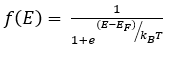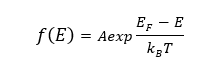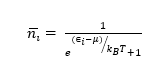# What is Fermi Dirac Distribution? Energy Band Diagram, and Boltzmann Approximation

Electrons and holes play an essential role in the transfer of electricity in semiconductors. These particles are arranged at a different energy level in a semiconductor. The movement of electrons from one energy level to other generates electricity.  An electron inside the metal should possess an energy level that is at least greater than the surface barrier energy to escape to a higher energy level.

There were many theses proposed and accepted explaining the characteristics and behavior of electrons. But some behavior of electron such as the independence of emission current on temperature etc… still remained a mystery. Then a breakthrough statistics, Fermi Dirac Statistics, published by Enrico Fermi and Paul Dirac in 1926 helped to solve these puzzles.

From then Fermi Dirac Distribution is being applied to explain the collapse of a star to a white dwarf, to explain free electron emission from metals etc….

## Fermi Dirac Distribution

Before getting into the Fermi Dirac Distribution function let us look at the energy distribution of electrons in various types of semiconductor. The maximum energy of a free electron can have in a material at absolute temperature .i.e. at 0k is known as Fermi energy level. The value of Fermi energy varies for different materials. Based on the energy possessed by electrons in a semiconductor, electrons are arranged in three energy bands – Conduction band, Fermi energy level, Valency band.

While conduction band contains excited electrons, valence band contains holes. But what does the Fermi level meant for? Fermi level is the energy state which has probability ½ of being occupied by an electron. In simple terms, it is the maximum energy level that an electron can have at 0k and the probability of finding the electron above this level at absolute temperature is 0. At absolute zero temperature, half of the Fermi level will be filled with electrons.

In energy band diagram of semiconductor, Fermi level lies in the middle of conduction and valence band for an intrinsic semiconductor. For extrinsic semiconductor, Fermi level lies near valency band in P-type semiconductor and for N-type semiconductor, it lies near to the conduction band.

Fermi energy level is denoted by EF, the conduction band is denoted as EC and valence band is denoted as EV.

Fermi level in N and P-type semiconductors

## Fermi Dirac Distribution Function

The probability that the available energy state ‘E’ will be occupied by an electron at absolute temperature T under conditions of thermal equilibrium is given by the Fermi-Dirac function. From quantum physics, the Fermi-Dirac Distribution Expression isWhere k is the Boltzmann constant in OK, T is the temperature in 0K and EF is the Fermi energy level in eV.k= 1.38X10-23 J/K

The Fermi level represents the energy state with a 50% probability of being filled if no forbidden band exists, .i.e., if E = EF then f(E)=1/2 for any value of temperature.

Fermi-Dirac distribution only gives the probability of occupancy of the state at a given energy level but doesn’t provide any information about the number of states available at that energy level.

### Fermi Dirac Distribution and Energy Band Diagram

The above plot shows the behavior of Fermi level at various temperature ranges T= 00K, T= 3000K, T= 25000K. At T=0K, the curve has step-like characteristics.

At T = 00K, the total number of energy levels occupied by electrons can be known by using the Fermi-Dirac Function.

For a given energy level E > EF, the exponential term in the Fermi-Dirac function becomes 0 and  Which means that the probability of finding the occupied energy level of energy greater than EF is zero.

For a given energy level E < EF the value of which means that all the energy levels with energy are less than that of Fermi level EF will be occupied at T = 00K.  This indicates that the Fermi energy level is the maximum energy an electron can have at absolute zero temperature.

For temperature greater than absolute temperature and E = EF, then independent of the value of temperature.

For temperature greater than absolute temperature and E < EF, then the exponential will be negative. f(E) starts at 0.5 and tends to increase towards 1 as E decreases.

For temperature greater than absolute temperature and E > EF, the exponential will be positive and increases with E. f(E) starts from 0.5 and tends to decrease towards 0 as E increases.

### Fermi Dirac Distribution Boltzmann Approximation

Maxwell- Boltzmann distribution is the commonly used Fermi Dirac distribution approximation.

Fermi-Dirac Distribution is given byBy using Maxwell– Boltzmann approximation the above equation is reduced toWhen the difference between the carrier’s energy and Fermi level is large compared to, the term 1 in the denominator can be neglected.  For the application of Fermi-Dirac distribution, the electron must follow Pauli’s exclusive principle, which is important at high doping. But Maxwell-Boltzmann distribution neglects this principle, thus Maxwell-Boltzmann approximation is limited to lowly doped cases.

### Fermi Dirac and Bose-Einstein Statistics

Fermi-Dirac statistics is the branch of quantum statistics, that describes the distribution of particles in energy states that contains identical particles obeying Pauli-Exclusion Principle. Since F-D statistics is applied to particles with half-integer spin, these are called fermions.

A system consisting of thermodynamically at equilibrium and  identical particles, in the single- particle state I, the average number of fermions is given by F-D distribution aswhere is the single-particle state I, the total chemical potential is denoted by, kB is the Boltzmann constant whereas T is the absolute temperature.

Bose-Einstein statistics is the opposite of F-D Statistics. This is applied to particles with full integer spin or no spin, called Bosons. These particles don’t obey the Pauli Exclusion Principle, which means that the same quantum configuration can be filled with more than one boson.

F-D statistics and Bore-Einstein statistics are applied when the quantum effect is important and the particles are indistinguishable.

### Fermi Dirac Distribution Problem

In a solid consider the energy level lying 0.11eV below the Fermi level. Find the probability of this level not being occupied by the electron?